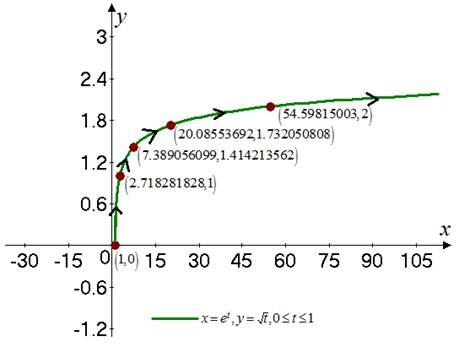# The sketch for the curve represented by the parametric equations and then indicate the direction in which the curve is traced as the value of t increases.### Single Variable Calculus: Concepts...

4th Edition
James Stewart
Publisher: Cengage Learning
ISBN: 9781337687805### Single Variable Calculus: Concepts...

4th Edition
James Stewart
Publisher: Cengage Learning
ISBN: 9781337687805

#### Solutions

Chapter 1, Problem 31RE

(a)

To determine

## To find: The sketch for the curve represented by the parametric equations and then indicate the direction in which the curve is traced as the value of t increases.

Expert Solution

### Explanation of Solution

Given:

The given parametric equations is x=et and y=t .

The range of the parameter t is 0t1 .

Calculation:

Consider the given parametric equations are,

x=ety=t

The table for the value of x and y for different value of the parameter is shown in Table 1

Table 1

 t x y 0 1 0 1 2.718281828 1 2 7.389056099 1.414213562 3 20.08553692 1.732050808 4 54.59815003 2 5 148.413591 2.236067977 6 403.4284935 2.44945743

The plot for the above points on the coordinate plane is shown in Figure 1Figure 1

(b)

To determine

### To find: The Cartesian equation of the curve.

Expert Solution

The required Cartesian equation is y=lnx .

### Explanation of Solution

Given:

The given parametric equations is x=et and y=t .

The range of the parameter t is 0t1 .

Calculation:

Consider the given parametric equations are,

x=ety=t

Take natural log on both the sides for the equation x=et as,

lnx=lnetlnx=t

Consider the equation y=t as,

y=lnx

Thus, the required Cartesian equation is y=lnx .

### Have a homework question?

Subscribe to bartleby learn! Ask subject matter experts 30 homework questions each month. Plus, you’ll have access to millions of step-by-step textbook answers!